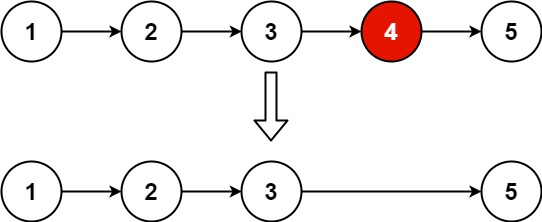### LeetCode• ㊗️
• 大家
• offer
• 多多！

## Problem

Given the head of a linked list, remove the $n^{th}$ node from the end of the list and return its head.

Example 1:Input: head = [1,2,3,4,5], n = 2
Output: [1,2,3,5]


Example 2:

Input: head = , n = 1
Output: []


Example 3:

Input: head = [1,2], n = 1
Output: 


Constraints:

• The number of nodes in the list is sz.
• 1 <= sz <= 30
• 0 <= Node.val <= 100
• 1 <= n <= sz

## Code

class Solution {
public ListNode removeNthFromEnd(ListNode head, int n) {
ListNode dummy = new ListNode(0);
ListNode first = dummy;
ListNode second = dummy;

for(int i = 0; i <= n; i++){
first = first.next;
}

while(first != null){
first = first.next;
second = second.next;
}

second.next = second.next.next;
return dummy.next;
}
}